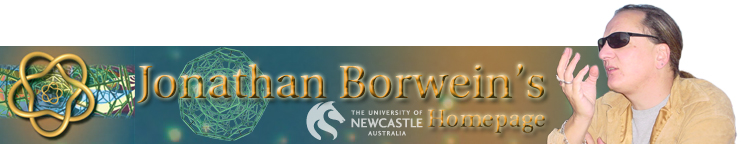## 2009: Introduction to Experimental Mathematics

Experimental Mathematics is the use of a computer to run computations - sometimes no more than trial-and-error tests - to look for patterns, to identify particular numbers and sequences, to gather evidence in support of specific mathematical assertions, assertions that may themselves arise by computational means, including search. Like contemporary chemists - and before them the alchemists of old - who mix various substances together in a crucible and heat them to a high temperature to see what would happen, today's experimental mathematician puts a hopefully potent mix of numbers, formulas, and algorithms into a computer in the hope that something of interest emerges.

This course will provide a rigorous introduction to Experimental Mathematics while also exploring a variety of pure and applied mathematical topics that the student may well not have seen during an undergraduate degree.

Course Goals The successful student will emerge from this course with much enhanced abilities to:

• Use current mathematical computation tools
• Learn mathematics independently
• Formulate and refine conjectures
• Develop strategies for proof or refutation of new mathematics

• Place and Time V120 from 1.00-3.00pm on Tuesdays starting on August 4th (with a backup time to be determined)

• Text J.M. Borwein and D.H. Bailey, Mathematics by Experiment: Plausible Reasoning in the 21st Century, Expanded 2nd edition, AK Peters, 2008.

• Assessment There will be four graded assignments each counting for 25% of the final mark. In each case you will be asked to select 10 exercises (with my approval) from chapters in the Text and produce full answers in LaTeX or similar form. The rough schedule is as follows:

1. Week 4 (Chapters 2 and 3)
2. Week 9 (Chapters 4 and 5)
3. Week 11 (Chapter 5 and 6)
4. Week 14 (Chapter 7)